## Libros Nuevos de Noviembre 2022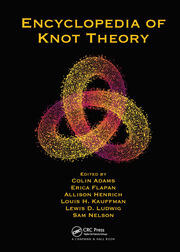Adams, Colin  (Ed.)
Encyclopedia of knot theory
Boca Raton, Florida : CRC Press, 2021. xi, 941 p.
QA612.2 E53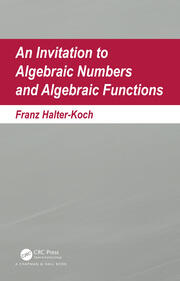Halter-Koch, Franz
An invitation to algebraic numbers and algebraic functions
Boca Raton, FL : CRC Press, 2020. xiv, 580 p.
QA247 H354Al-Baali, Mehidden (Ed.)
Numerical analysis and optimization
Cham, Switzerland : Springer, 2021. xviii, 297 p. (Springer proceedings in mathematics & statistics ; vol. 354)
QA297 I574 2020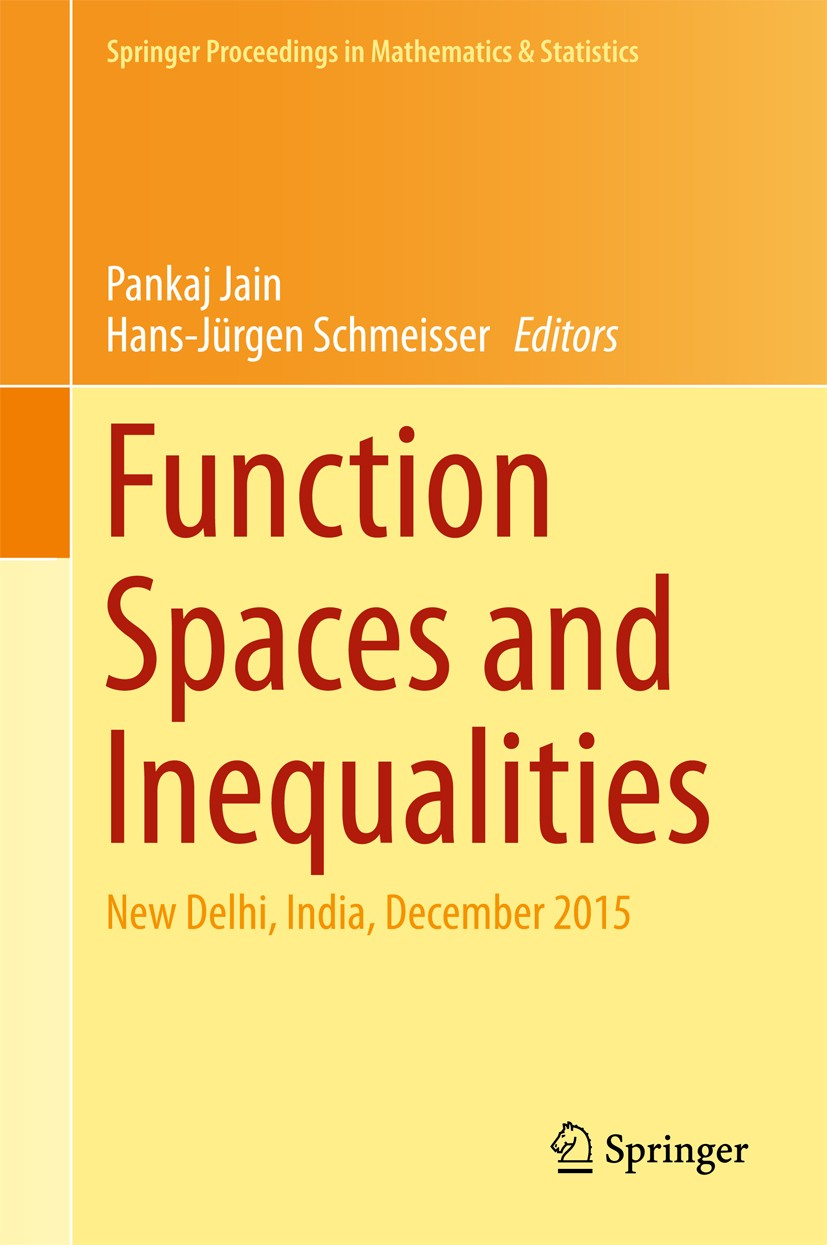Jain, Pankaj (Ed.)
Function spaces and inequalities
Singapore : Springer Nature, 2017. viii, 335 p. -- (Springer Proceedings in Mathematics & Statistics ; vol. 206)
QA403 F858Andrews, George E.
Analytic number theory, modular forms and q-hypergeometric series
Cham, Switzerland : Springer, 2017. 731 p. -- (Springer proceedings in mathematics & statistics)
QA241 A542Ay, Nihat (Ed.)
Information geometry and its applications
Cham, Switzerland : Springer, 2018. xi, 456 p. -- (Springer proceedings in mathematics & statistics ; vol. 252)
QA10.4 I57 2016Baigent, Steve
Progress on difference equations and discrete dynamical systems
Cham, Switzerland : Springer, 2020.  ix, 448 p. -- (Springer proceedings in mathematics & statistics ; vol. 341)
QA431 I58 2019Barlow, Martin T.
Random graphs, phase transitions, and the gaussian free field
Cham, Switzerland : Springer : 2020. xvii, 407 p. --  (Springer proceedings in Mathematics & statistics ; vol. 304)
QA273.A1 P55 2017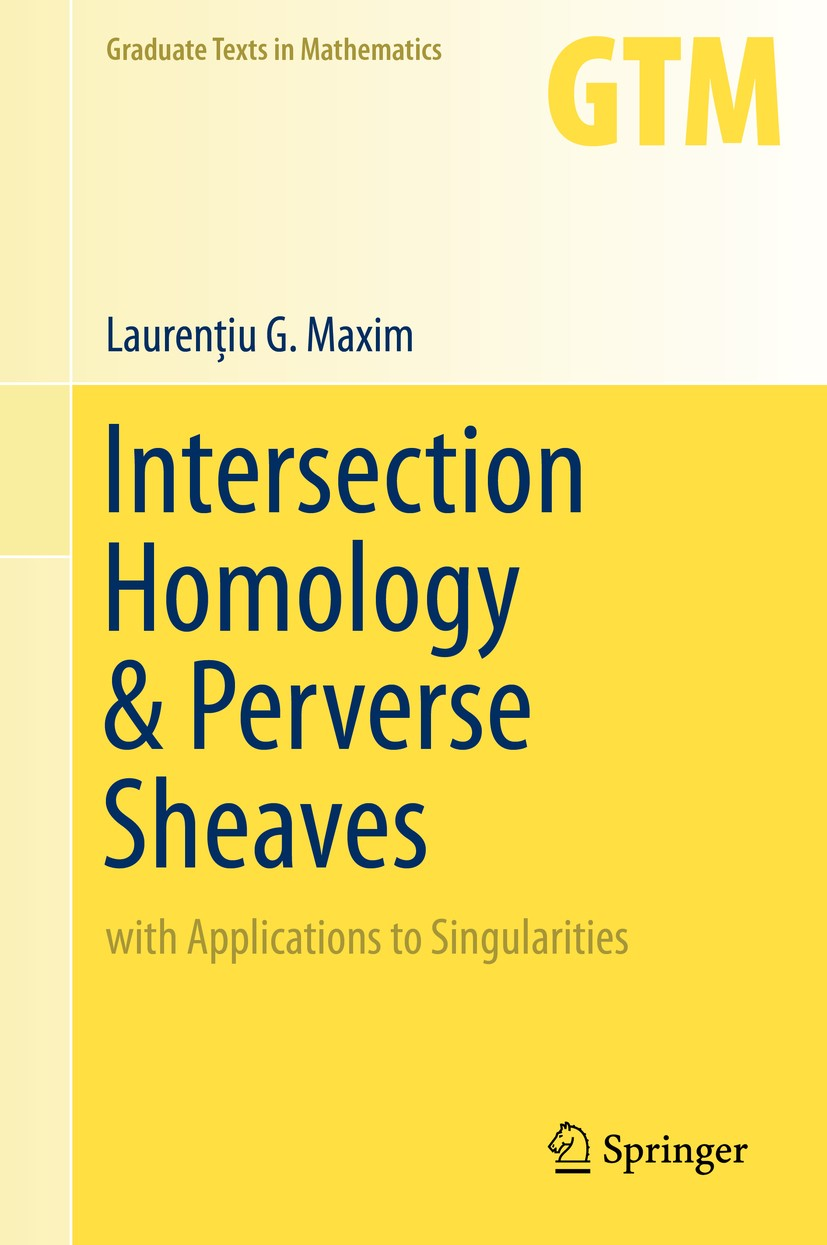Maxim, Laurenţiu G.
Intersection homology & perverse sheaves : with applications to singularities
Cham : Springer, 2019. xv, 270 p. -- (Graduate texts in mathematics ; vol. 281)
QA612.32 M39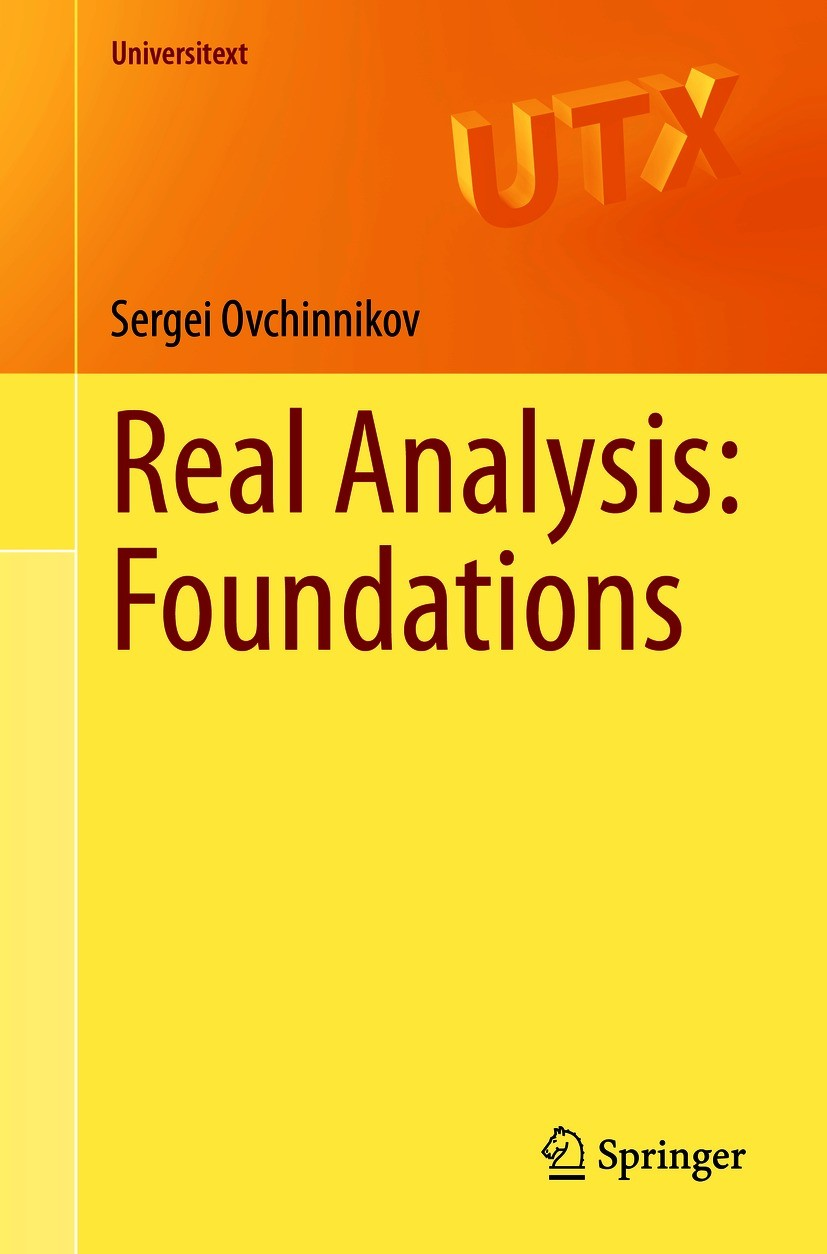Ovchinnikov, Sergei
Real analysis : foundations
Cham, Switzerland : Springer, 2021. xii, 178 p. -- (Universitext)
QA331.5 O83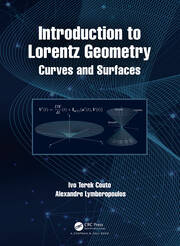Couto, Ivo Terek
Introduction to Lorentz geometry : curves and surfaces
Boca Raton, Florida : CRC Press, 2021. ix, 340 p.
QA641 C69Plymen, Roger J.
The great prime number race
Providence, Rhode Island : American Mathematical Society, 2020. xii, 138 p. -- (Student mathematical library ; vol. 92)
QA246 P585Reis, Timo  (Ed.)
Progress in differential-algebraic equations II
Cham, Switzerland : Springer, 2020. xx, 486 p. -- (Differential-algebraic equations forum)
QA372.5 P75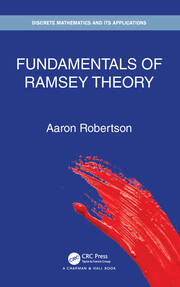Robertson, Aaron
Fundamentals of Ramsey theory
Boca Raton : Chapman & Hall, CRC Press, 2021. xiii, 241 p. -- (Discrete mathematics and its applications)
QA166 R69Davis, Diana (Ed.)
Illustrating mathematics
Providence, Rhode Island : American Mathematical Society, 2020. iii, 171 p.
QA93 I55Scheepers, Marion (Ed.)
Centenary of the Borel conjecture
Providence, Rhode Island : American Mathematical Society, 2020. viii, 242 p. -- (Contemporary mathematics ; vol. 755)
QA248 C45Deines, Alyson (Ed.)
Cham, Switzerland : Springer, 2018. xiv, 270 p. -- (Association for women in mathematics series ; vol. 15)
QA1 A38Schmüdgen, Konrad
An invitation to unbounded representations of *-algebras on Hilbert space
Cham, Switzerland : Springer, 2020.  xviii, 381 p. -- (Graduate texts in mathematics ; vol. 285)
QA322.4 S34Elaydi, Saber (Ed.)
Advances in difference equations and discrete dynamical systems
Singapore : Springer, 2017. x, 282 p. -- (Springer proceedings in mathematics & statistics ; vol. 212)
QA431 I58 2016Stanley, Richard P.
Conversational problem solving
Providence, Rhode Island : American Mathematical Society, 2020. ix, 178 p.
QA63 S73Feldvoss, Jorg (Ed.)
Switzerland : Springer, 2019. xviii, 322 p. -- (Springer proceedings in mathematics & statistics ; vol. 277)
QA150 S68

Hermitian-Grassmannian submanifolds
Singapore : Springer, 2017. xi, 360 p. -- (Springer proceedings in mathematics & statistics ; vol. 203)
QA649 I574 2016Garg, Harish (Ed.)
Pythagorean fuzzy sets : theory and applications
Singapore : Springer, 2021. xi, 442 p.
QA248.5 P97Wood, David R.
2019-20 MATRIX annals
Cham, Switzerland : Springer, 2021. xliv, 803 p. -- (MATRIX book series ; vol. 4)
QA11.2 M375 2021

### ¿Quién está en línea?

Hay 83 invitados y un miembro en línea

Go to top